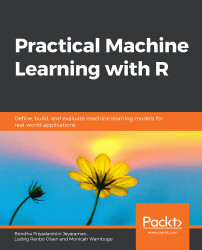•#### Practical Machine Learning with R#### Overview of this book

With huge amounts of data being generated every moment, businesses need applications that apply complex mathematical calculations to data repeatedly and at speed. With machine learning techniques and R, you can easily develop these kinds of applications in an efficient way. Practical Machine Learning with R begins by helping you grasp the basics of machine learning methods, while also highlighting how and why they work. You will understand how to get these algorithms to work in practice, rather than focusing on mathematical derivations. As you progress from one chapter to another, you will gain hands-on experience of building a machine learning solution in R. Next, using R packages such as rpart, random forest, and multiple imputation by chained equations (MICE), you will learn to implement algorithms including neural net classifier, decision trees, and linear and non-linear regression. As you progress through the book, you’ll delve into various machine learning techniques for both supervised and unsupervised learning approaches. In addition to this, you’ll gain insights into partitioning the datasets and mechanisms to evaluate the results from each model and be able to compare them. By the end of this book, you will have gained expertise in solving your business problems, starting by forming a good problem statement, selecting the most appropriate model to solve your problem, and then ensuring that you do not overtrain it.Free Chapter
An Introduction to Machine LearningData Cleaning and Pre-processingFeature EngineeringIntroduction to neuralnet and Evaluation MethodsLinear and Logistic Regression ModelsUnsupervised LearningAppendix## Handling Missing Values, Duplicates, and Outliers

In any dataset, we might have missing values, duplicate values, or outliers. We need to ensure that these are handled appropriately so that the data used by the model is clean.

### Handling Missing Values

Missing values in a data frame can affect the model during the training process. Therefore, they need to be identified and handled during the pre-processing stage. They are represented as NA in a data frame. Using the example that follows, we will see how to identify a missing value in a dataset.

Using the is.na(), complete.cases(), and md.pattern() functions, we will identify the missing values.

The is.na() function, as the name suggests, returns TRUE for those elements marked NA or, for numeric or complex vectors, NaN (Not a Number) , and FALSE. The complete.cases() function returns TRUE if the value is missing and md.pattern() gives a summary of the missing values.

### Exercise 12: Identifying the Missing Values

In the following example, we are adding...